Letbe a differential function with g(0) = 0, ...

### Related TestLet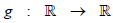be a differential function with g(0) = 0, g'(0) = 0 and g'(1)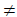0.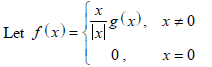and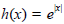for all x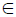R. Let (f o h)(x) denote f(h(x)) and (h o f)(x) denote h(f(x)). Then which of the following is (are) true?
• a)
f is differentiable at x = 0
• b)
h is differentiable at x = 0
• c)
f o h is differentiable at x = 0
• d)
h o f is differentiable at x = 0Atmi Devi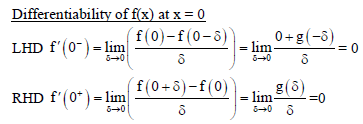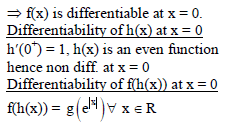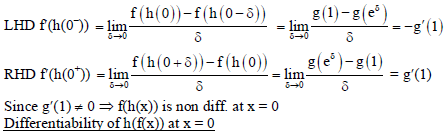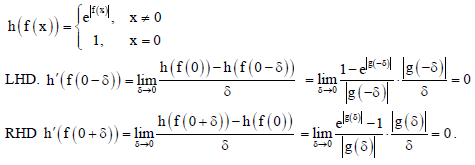View courses related to this question Explore JEE courses
 Explore JEE coursesView courses related to this question1 Crore+ students have signed up on EduRev. Have you?

• ### Let f : R -->R be a differentiable function with f (0) = 0. If y = f (x)... more(Scan QR code)